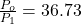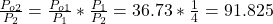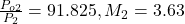## For a given Prandtl-Meyer expansion, the upstream Mach number is 3 and the pressure ratio across the wave is P2/P1 = 0.4. Calculate teh angl

Question

For a given Prandtl-Meyer expansion, the upstream Mach number is 3 and the pressure ratio across the wave is P2/P1 = 0.4. Calculate teh angles of the forward and rearward mach lines of the expansion fan relative to the free-stream direction

in progress 0
2 months 2021-07-21T12:17:05+00:00 1 Answers 3 views 0

The angle for the forward Mach line is 19.47°

The angle for the rearward Mach line is 5.21°

Explanation:

From table A-1 (Modern Compressible Flow: with historical perspective):(M₁ = 3)

If Po₁ = Po₂Table A-1:Table A-5:

v₁ = 49.76°

μ₁ = 19.47°

v₂ = 60.55°

μ₂ = 16°

θ = 60.55 – 49.76 = 10.79°

The angle for the forward Mach line is:

μ₁ = 19.47°

The angle for the rearward Mach line is:

θr = μ₂ – θ = 16 – 10.79 = 5.21°